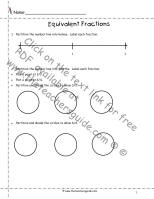# Fractions Worksheets

###Fractions Equal Parts

Students determine if a shape is divided into equal parts.

###Fractions How Many Equal Parts

Students determine how many equal parts the shape has.

###Fractions Unit Fractions

Students determine the unit fractions.

###Fractions What Fraction is Shaded

Students determine the fraction shaded.

###Fractions Shade in the Fraction

Students shade in the fraction.

###Fractions Partition the Shape to Shade in the Fraction

Students partition the shape to shade in the correct fraction.

###Fractions Shade More than one Whole

Students shade in fractions that show more than one whole.

###Fractions on the Numberline 1

Students show the fractions on the numberline.

###Fractions on the Numberline 2

Students show the fractions on the numberline.

###Fractions on the Numberline 3

Students show the fractions on the numberline.

###Fractions on the Numberline 4

Students show the fractions on the numberline.

###Equivalent Fractions

Students make equivalent fractions.

###Equivalent Fractions by Multiplying

Students make equivalent fractions.

###Fractions Dominoes

Students use dominoes to create fractions.